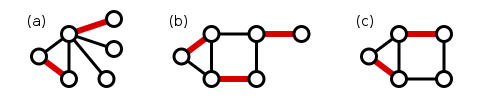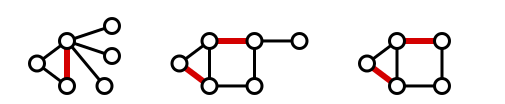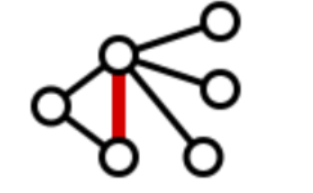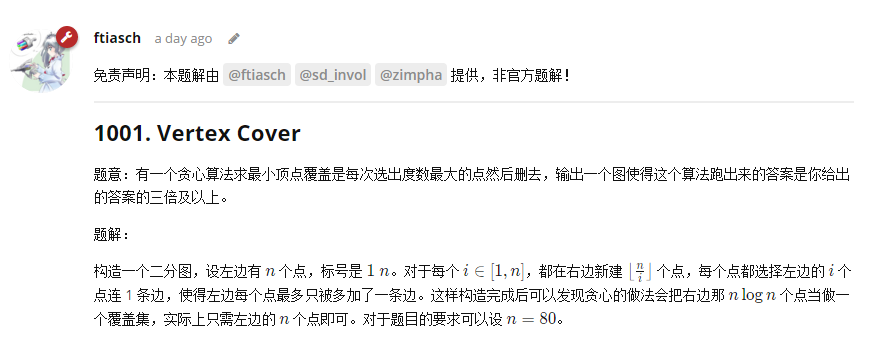• 问题描述：就是在图中找最小的点集，使得覆盖所有边。 和独立集等价：独立集问题：在图中找最大的点集，使得点集内的所有点互不相连。 引理：顶点覆盖集和独立集互补。 ...上面这个引理使得这两个问题可以相互规约...

问题描述：就是在图中找最小的点集，使得覆盖所有边。
和独立集等价：独立集问题：在图中找最大的点集，使得点集内的所有点互不相连。

引理：顶点覆盖集和独立集互补。

上面这个引理使得这两个问题可以相互规约，从而这两个问题等价。

等价问题：给定图G和数k, 问G包含大小至少为k的独立集吗？
为什么等价：如果我们能在多项式时间内给出这个问题的答案，那么我们可以通过二分查找得到最大独立集的size为K。一个点如果是最大独立集中的点，等价于除去这个点后得到的图G'含有一个K-1大小的独立集。那么我们每次选取一个顶点v，然后再问一下G'是否有K-1的独立集，如果回答为yes，那么我们把v加入我们的答案中，如果回答为no, 我们把v的邻居加入答案中（因为如果v不在答案中，v的邻居也不在答案中，那么v和邻居之间的边将不会被覆盖）。所以，如果上述提问的解法是多项式的，那么最大独立集问题也是多项式的。

转载于:https://www.cnblogs.com/huangshiyu13/p/7044196.html
展开全文• vertex coverapproximation factor（近似比）maximum matching&&minimum vertex covermaximum matching（最大匹配）vertex cover（点覆盖） approximation factor（近似比） 近似比是近似算法所得的近似解与...


创新实训个人记录：approximation factor, maximum matching&&vertex cover
approximation factor（近似比）maximum matching&&minimum vertex covermaximum matching（最大匹配）vertex cover（点覆盖）
approximate algorithm on vertex cover（点覆盖问题的近似算法）approximate algorithm（近似算法）先找OPT下界：极大匹配提供了点覆盖问题的下界近似算法
approximate factor（近似因子）

approximation factor（近似比）
近似比是近似算法所得的近似解与最优解OPT的比值。我们想用近似算法找到NP问题的近似解，需要通过近似比来论证近似算法的优秀。但是，对于NP问题，我们找不到OPT，又如何得到近似比呢？ 这里涉及到近似算法中求近似比的通用原则，让我们不知道OPT也可以求近似比，即先求OPT的下界，再找到一个近似算法，要求这个算法能求得与下界成常数倍关系的近似解。比如，OPT≥a，c·OPT≥c·a；那么我们需要找到一个近似算法求得近似解c·a，此时有c·a/OPT≤c·OPT/OPT=c，我们称近似比为c。
maximum matching&&minimum vertex cover
maximum matching（最大匹配）
matching：匹配。给定一个图H =(U,F)，一个边集M ⊆ F，如果M中没有两条边共享一个端点，即M中任意两条边都不交于一个点，那么M被称为是一个匹配。 maximum matching：最大匹配。最大基数匹配，即这样的匹配可以找到的匹配边数是最多的。maximal matching：极大匹配。我们不能再找到图H中的任何一条边，添加到匹配中仍满足匹配条件。vertex cover（点覆盖）
给定一个无向图G=(V,E), 有一个成本函数：V→ Q+，找到最小成本点覆盖，即一个集合V’⊆ V，对于V’，G中每条边至少有一个端点在V’中，这样的V’就是一个点覆盖，我们要找到最小的点集V’，即最小成本点覆盖。在所有点都是单位成本的情况下，我们称点覆盖问题为基数点覆盖问题。（这本书定义的“点覆盖”直接是最小成本点覆盖，这个问题还没有被证明是NP问题。）
approximate algorithm on vertex cover（点覆盖问题的近似算法）
approximate algorithm（近似算法）
先找OPT下界：极大匹配提供了点覆盖问题的下界
极大匹配：如果再加任意一条边到匹配中，那么一定会有至少一条边与新加入的边交于一个点。 下界：任何的点覆盖都不得不至少选择每个极大匹配边的一个端点。
我们按照极大匹配把一个图分成匹配边和未匹配边，那么任意一个点覆盖必定包含匹配边的一个端点，不然这个点覆盖就无法覆盖这个匹配边，那么就不是一个点覆盖。如果不是所有匹配边和未匹配边都交于一个点的话，点覆盖还需要包含未匹配边的端点，那么点覆盖的点集的点的数目必定是大于等于极大匹配的边集的边的数目。（如下图，即不是匹配边和未匹配边都交于一个点。）理所当然地，也大于等于非极大匹配的边集的边的数目。所以，我们有：所有的匹配对应的边集中边的数目总是小于等于所有的点覆盖对应的点集中点的数目，匹配的边集的边数是点覆盖的点集的点数的下界。更紧地，极大匹配的边集的边数是最小点覆盖的点集的点数的下界。
近似算法
找到图G中的一个极大匹配，输出极大匹配的点集。
approximate factor（近似因子）
近似比为2。 也就是说，该近似算法所得出的解是个可行解，而且这个可行解的成本一定在这个问题的最优解的成本的2倍之内，也就是我们要证极大匹配的点集（极大匹配边的左右端点），一定在最小基数点覆盖的点集的2倍内。
证明：
如果是极大匹配的点集，那么一定是覆盖了所有边的至少一个端点，否则的话，可以再加一条没有被覆盖的边进入匹配中成为更大的匹配。我们现在让M作为极大匹配所选择的边集。之前我们已推出 |M|≤OPT，即所有的匹配对应的边集中边的数目总是小于等于所有的点覆盖对应的点集中点的数目，最大的匹配中边的数目≤最小点覆盖中点的数目。Kőnig’s theorem: 在二分图中，最大的匹配的边集中边的数目等于最小点覆盖的点集中点的数目）我们观察得，这种算法选择的覆盖，由于匹配的边为|M|,每条边左右两个端点，那么对应的覆盖点数为2|M|,因为|M|≤OPT，所以2|M|≤2·OPT，即依靠下界方案得到的最大的匹配的点集（匹配边的左右端点）≤最小点覆盖的点集的2倍/最优解的2倍，最大的匹配的点集/最小点覆盖的点集≤2。
展开全文算法
• Vertex Cover Time Limit: 2000/1000 MS (Java/Others) Memory Limit: 256000/256000 K (Java/Others) Total Submission(s): 421 Accepted Submission(s): 162 Special Judge Problem Description

Vertex Cover
Time Limit: 2000/1000 MS (Java/Others)    Memory Limit: 256000/256000 K (Java/Others) Total Submission(s): 421    Accepted Submission(s): 162 Special Judge

Problem Description

As we know,

minimumvertexcover
is a classical NP-complete problem. There will not be polynomial time algorithm for it unless

P=NP
.

You can see the definition of vertex cover in https://en.wikipedia.org/wiki/Vertex_cover.

Today, little M designs an "approximate" algorithm for vertex cover. It is a greedy algorithm. The main idea of her algorithm is that always choosing the maximum degree vertex into the solution set. The pseudo code of her algorithm is as follows:

We assume that the labels of the vertices are from 1 to n.

for (int i = 1; i <= n; ++i) {
use[i] = false;
deg[i] = degree of the vertex i;
}
int ans = 0;
while (true) {
int mx = -1, u;
for (int i = 1; i <= n; ++i) {
if (use[i])
continue;
if (deg[i] >= mx) {
mx = deg[i];
u = i;
}
}
if (mx <= 0)
break;
++ans;
use[u] = true;
for (each vertex v adjacent to u)
--deg[v];
}
return ans;

As a theory computer scientist, you immediately find that it is a bad algorithm. To show her that this algorithm dose not have a constant approximate factor, you need to construct an instance of vertex cover such that the solution get by this algorithm is much worse than the optimal solution.

Formally, your program need to output a simple undirected graph of at most

500
vertices. Moreover, you need to output a vertex cover solution of your graph. Your program will get Accept if and only if the solution size get by the above algorithm is at least three times as much as yours.

Input

There is no input.

Output

First, output two integer

n
and

m
in a line, separated by a space, means the number of the vertices and the number of the edges in your graph.
In the next

m
lines, you should output two integers

u
and

v
for each line, separated by a space, which denotes an edge of your graph. It must be satisfied that

1≤u,v≤n
and your graph is a simple undirected graph.
In the next line, output an integer

k(1≤k≤n)
, means the size of your vertex cover solution.
Then output

k
lines, each line contains an integer

u(1≤u≤n)
which denotes the label of a vertex in your solution. It must be satisfied that your solution is a vertex cover of your graph.

Sample Output

4 4
1 2
2 3
3 4
4 1
2
1
3

Hint

The sample output is just to exemplify the output format.

题目大意：

求最小点覆盖，主人公想到了一个贪心的方法（方法在题干中用伪代码写出了）；
我们需要找到一组数据hack他，使得他的解是正解的至少三倍。

思路：

按照ICPCCamp给出的题解写的：Ac代码：

#include<stdio.h>
#include<string.h>
using namespace std;
struct node
{
int x,y;
}ans;
int main()
{
int cnt=0;
int L=20;
int R=20;
for(int i=1;i<=L;i++)
{
for(int j=1;j<=L;j+=i)
{
if(j+i-1>L)break;
R++;
for(int k=j;k<=j+i-1;k++)
{
ans[cnt].x=k;
ans[cnt].y=R;
cnt++;
}
}
}
printf("%d %d\n",R,cnt);
for(int i=0;i<cnt;i++)
{
printf("%d %d\n",ans[i].x,ans[i].y);
}
printf("%d\n",L);
for(int i=1;i<=L;i++)
{
printf("%d\n",i);
}
}


展开全文• Vertex Cover Time Limit: 2000/1000 MS (Java/Others) Memory Limit: 256000/256000 K (Java/Others) Total Submission(s): 0 Accepted Submission(s): 0 Special Judge Problem Description As we kn
Vertex Cover
Time Limit: 2000/1000 MS (Java/Others)    Memory Limit: 256000/256000 K (Java/Others) Total Submission(s): 0    Accepted Submission(s): 0 Special Judge

Problem Description

As we know,

minimumvertexcover
is a classical NP-complete problem. There will not be polynomial time algorithm for it unless

P=NP
.

You can see the definition of vertex cover in https://en.wikipedia.org/wiki/Vertex_cover.

Today, little M designs an "approximate" algorithm for vertex cover. It is a greedy algorithm. The main idea of her algorithm is that always choosing the maximum degree vertex into the solution set. The pseudo code of her algorithm is as follows:

We assume that the labels of the vertices are from 1 to n.

for (int i = 1; i <= n; ++i) {
use[i] = false;
deg[i] = degree of the vertex i;
}
int ans = 0;
while (true) {
int mx = -1, u;
for (int i = 1; i <= n; ++i) {
if (use[i])
continue;
if (deg[i] >= mx) {
mx = deg[i];
u = i;
}
}
if (mx <= 0)
break;
++ans;
use[u] = true;
for (each vertex v adjacent to u)
--deg[v];
}
return ans;

As a theory computer scientist, you immediately find that it is a bad algorithm. To show her that this algorithm dose not have a constant approximate factor, you need to construct an instance of vertex cover such that the solution get by this algorithm is much worse than the optimal solution.

Formally, your program need to output a simple undirected graph of at most

500
vertices. Moreover, you need to output a vertex cover solution of your graph. Your program will get Accept if and only if the solution size get by the above algorithm is at least three times as much as yours.

Input

There is no input.

Output

First, output two integer

n
and

m
in a line, separated by a space, means the number of the vertices and the number of the edges in your graph.
In the next

m
lines, you should output two integers

u
and

v
for each line, separated by a space, which denotes an edge of your graph. It must be satisfied that

1≤u,v≤n
and your graph is a simple undirected graph.
In the next line, output an integer

k(1≤k≤n)
, means the size of your vertex cover solution.
Then output

k
lines, each line contains an integer

u(1≤u≤n)
which denotes the label of a vertex in your solution. It must be satisfied that your solution is a vertex cover of your graph.

Sample Output

4 4
1 2
2 3
3 4
4 1
2
1
3

Hint

The sample output is just to exemplify the output format.


展开全文• The maximum balanced biclique problem (MBBP) is an important extension of the maximum clique problem (MCP), which has wide industrial applications. In this paper, we propose a new local search ...
• We call the maximum amount by which we can increase the flow on each edge in an augmenting path p the residual capacity of p ,given by Cuts of flow networks The flow is maximum if and only if its ...
• 正解:dp 解题报告: 这儿是传送门 又是个神仙题趴QAQ 这题就直接说解法辣?主要是思想比较难,真要说有什么不懂的知识点嘛也没有,所以也就没什么好另外先提一下的知识点QAQ ...首先取反,就变成了求最大独立集,就方便求...
• Maximum clique is the clique that has maximum number of vertex. Input Input contains multiple tests. For each test: The first line has one integer n, the number of vertex. (1 ) The following n ...each lines
• Maximum White Subtree time limit per test2 seconds memory limit per test256 megabytes inputstandard input outputstandard output You are given a tree consisting of n vertices. A tree is a connected ...
• As we know,minimumvertexcoverminimumvertexcoveris a classical NP-complete problem. There will not be polynomial time algorithm for it unlessP=NPP=NP. You can see the definition of vertex cover in ...构造
• Given a simple graph G with n vertices and a vertex cover C of G, if C has no removable vertices, output C. Else, for each removable vertex v of C, find the number ρ(C−{v}) of removablegraph
• ArrayList<Vertex> removeNbrs(ArrayList<Vertex> arlFirst, Vertex v) { ArrayList<Vertex> arlHold = new ArrayList<Vertex>(arlFirst); arlHold.removeAll(v.getNbrs()); return arlHold; } // ...最大团 图算法
• Texture matrix for texture unit n.The maximum dimension of the array is gl_MaxTureCoords. gl_NormalMatrix mat3 Inverse-transpose of the upper 3*3 of the modelview matrix gl_...VS 数据流
• ## OpenGL Vertex Array

千次阅读 2012-11-15 11:38:32
原文地址 http://www.songho.ca/opengl/gl_vertexarray.html OpenGL Vertex Array ...Related Topics: Vertex Buffer Object, Display ...Download: vertexArray.zip, vertexArray2.zip Update: Since
• The main idea of her algorithm is that always choosing the maximum degree vertex into the solution set. The pseudo code of her algorithm is as follows: We assume that the labels of the vertices ...acm 构造
• A tournament can be represented by a complete graph in which each vertex denotes a player and a directed edge is from vertex x to vertex y if player x beats player y. For a player x in
• } } } void Print(Vertex Start,Vertex End) { if(path[Start][End]==-1) return; else { Vertex k = path[Start][End]; Print(Start,k); printf("%d %d\n",C[k].X,C[k].Y); Print(k,End); } } ...数据结构 c++ 算法 c语言
• F. Maximum Weight Subset time limit per test 2 seconds memory limit per test 256 megabytes input standard input output standard output You are g...
• You are given a tree consisting of n vertices. A tree is a connected undirected graph with n−1 ... Each vertex v of this tree has a color assigned to it (av=1 if the vertex v is white and 0 if the ...
• For each special vertex, find another special vertex which is farthest from it (in terms of the previous paragraph, i.e. the corresponding distance is maximum possible) and output the distance between...
• Maximum Clique Time Limit: 20000/10000 MS (Java/Others) Memory Limit: 65536/32768 K (Java/Others) Total Submission(s): 92 Accepted Submission(s): 60 Problem Descripti...
•  了解这个算法是源于在Network Alignment问题中，图论算法用得比较多，而对于alignment，特别是pairwise alignment， 又经常遇到maximum bipartite matching问题，解决这个问题，是通过Network Flow问题的解法来...算法 c++ algorithm 匈牙利算法
• 解题报告 之 POJ 2699 The Maximum Number of Strong Kings 最大流 二分 枚举 建图
• Vertex Cover  Time Limit: 2000/1000 MS (Java/Others) Memory Limit: 256000/256000 K (Java/Others)
• As we know, minimumvertexcover is a classical NP-complete problem. There will not be polynomial time algorithm for it unless P=NP. You can see the definition of vertex cover in https
• Vertex Shader Built-In Variables The
• 原文地址：... 这是一篇openGL官方网站上的文章，比较清晰的讲述了Vertex Attributes的内容。 Vertex Attributes Introduction Vertex attributes are used t...